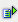The function rept (string, num) repeats a text string (string) as often as (num).

Syntax: =rept(string,num)Example Cell A1 = abc Cell A2 = 3 =rept(A1,A2) is abcabcabc